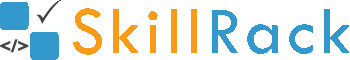ProgramID- 11202SkillRack
Armstrong Number

The program must accept an integer N as the input. The program must print yes if the integer N is an Armstrong number. Else the program must print no as the output. An X digit integer is said to be an Armstrong number or Narcissistic number if the sum of each digit raised to the power X is equal to the same integer.

Boundary Condition(s):
1 <= N <= 10^8

Input Format:
The first line contains N.

Output Format:
The first line contains either yes or no.

Example Input/Output 1:
Input:
1634

Output:
yes

Explanation:
The number of digits is 4.
1^4 + 6^4 + 3^4 + 4^4
= 1 + 1296 + 81 + 256
= 1634

Example Input/Output 2:
Input:
250

Output:
no

Explanation:
The number of digits is 3.
2^3 + 5^3 + 0^3
= 8 + 125 + 0
= 133

Max Execution Time Limit: 500 millisecs